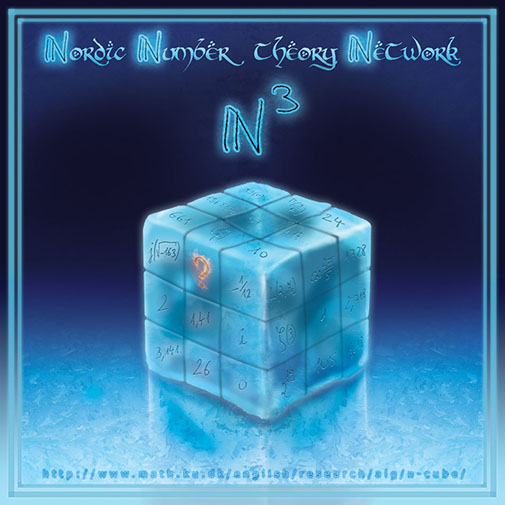$\mathbb{N}$ordic$\mathbb{N}$umber-theory$\mathbb{N}$etwork

The$\mathbb{N}$-cube network aims at developing number theory in many of its various aspects, from algebraic geometry and representation theory to computational problems, analytic number theory as well as diophantine geometry, moduli spaces properties or metric properties of numbers.

We are based in Northern Europe, working in different Universities. We sometimes have research guests in common and we organize the$\mathbb{N}$-cube Days on a regular basis.